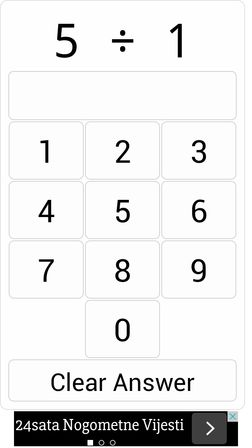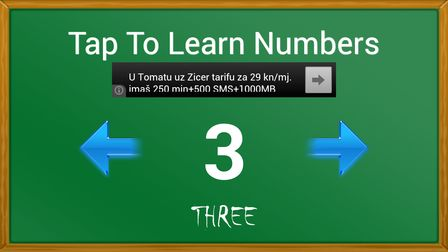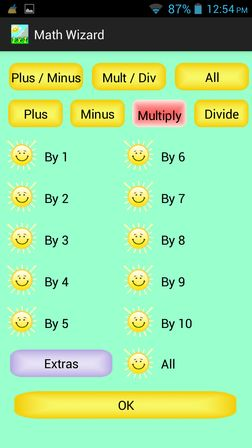Editor Ratings:
User Ratings:
[Total: 0 Average: 0]

Here’s a list of 5 math learning apps for Android which can be used by kids of all ages and even college students to brush up on their math knowledge and skills. Some people need more practice, some less to become good in math. With practice students who have problems solving math problems will be able to better understand the theory behind it all and hopefully they’ll be able to connect the dots better in the future when solving math problems.

Let’s look at these apps.

##(adsbygoogle = window.adsbygoogle || []).push({});

Math Workout is suitable for everyone, not just kids. In Math Workout, your goal is to guess as many of the 20 questions that you’re given correctly in as little time as possible.

App starts off with relatively easy basic arithmetic questions, but as you progress through it, you’ll be able to open up more advanced problems. By connecting online, you can share your results and compare with other Math Workout users.

Get Math Workout.

##Kids Math is an app that can help kids learn how to count and it also has training for basic math operation like subtraction, addition, multiplication and division.

When learning how to count, app first shows the number and then it’s read out loud and clear so that kids can repeat and memorize the numbers. When practicing math operations, they need to select the correct answer from the multiple choice options offered on the screen.

Get Kids Math.

Also have a look at Free Math Learning Software To Practice Mental Maths: QMentat.

##(adsbygoogle = window.adsbygoogle || []).push({});

Some kids, or should I say most kids, have problems learning multiplication tables. Math Tables is a math learning app for Android which specializes in multiplication tables.

There are three work modes. First is the practice mode where kids can learn multiplication tables, then there’s the puzzle mode and lastly the quiz mode which can be seen on the image above. In quiz mode, the correct answer needs to be selected from the given multiple choice answers.

Get Math Tables.

##Kids Learning Math Lite can help you kids learn how to count, how to subtract and how to add numbers. Paid version of the app unlocks more lessons.

App has nice looking, kids oriented user interface. All the numbers are read so that kids can learn them as they progress through the math problems. Same thing is of course, true when kids are learning how to count.

Also have a look at Help Kids Learn Math Uisng BuzzMath: Math Learning Website.

##Math Wizard is completely free math learning app where there aren’t any kind of restrictions when it comes to lesson availability.

It has lots of lessons available free of charge, subtraction, addition, multiplication, division and it also allows the little ones to learn their multiplication tables. Interface looks quite nice.

## Conclusion

Learning math is super important, and every little helps. From the list of apps above, I really like Math Wizard 101 because it has the largest selection of lessons. Try it, try them all and let me know what you think in comments down below.

 Editor Ratings: User Ratings:[Total: 0 Average: 0] Works With: Android Free/Paid: Free Tags: androidMath learning